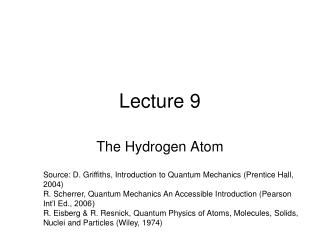DownloadDownload PresentationLecture 9

# Lecture 9

Télécharger la présentation## Lecture 9

- - - - - - - - - - - - - - - - - - - - - - - - - - - E N D - - - - - - - - - - - - - - - - - - - - - - - - - - -
##### Presentation Transcript

1. Lecture 9 The Hydrogen Atom Source: D. Griffiths, Introduction to Quantum Mechanics (Prentice Hall, 2004) R. Scherrer, Quantum Mechanics An Accessible Introduction (Pearson Int’l Ed., 2006) R. Eisberg & R. Resnick, Quantum Physics of Atoms, Molecules, Solids, Nuclei and Particles (Wiley, 1974)

2. Topics Today • Spherical Harmonics and Quantum Numbers • Probability of Finding Electron in a given volume • Most probable radius of hydrogen atom • Expectation value of radius of hydrogen atom • Angular Momentum • Angular Momentum Operators

3. Problem 1

4. Quantum Numbers

5. Problem 3

6. Probability of Finding Electron in a given Volume

7. The Most Probable RadiusHydrogen Ground State

8. Dropping off the constant terms and taking the derivative with respect to r and setting it equal to zero gives the radius for maximum probability. which gives where The most probable radius is the ground state radius obtained from the Bohr theory. The Schrodinger equation confirms the first Bohr radius as the most probable radius but goes further to describe in detail the profile of probability for the electron radius.

9. Spherical Harmonics

10. Radial Wave Function of Hydrogen Atom

11. Hydrogen 2s Radial Probability

12. Hydrogen 2p Radial Probability

13. Hydrogen 3s Radial Probability

14. Hydrogen 3p Radial Probability

15. Hydrogen 3d Radial Probability

16. The Expectation Value for RadiusHydrogen Ground State This requires integration by parts. The solution is All the terms containing r are zero, leaving It may seem a bit surprising that the average value of r is 1.5 x the first Bohr radius, which is the most probable value. The extended tail of the probability density accounts for the average being greater than the most probable value.

17. Probability for a Range of RadiusHydrogen Ground State This requires integration by parts. The form of the solution is

18. Problem 2 Problem 4.44 (Griffith) – n, l and m values are changed.

19. Angular Momentum Operators Lx and Ly do not commute: Use: =0

20. Angular Momentum Operators The non-commutativity of these operators means that in general no two components of L can be known simultaneously with infinite precision. (The only exception is that they can all be zero simultaneously.)

21. Generalized Uncertainty Principle